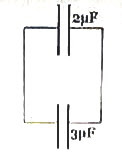## Tuesday, February 05, 2008

### Electrostatics- Multiple Choice Questions Involving Capcitors

The following questions will help you to understand the calculation of common potential difference and the energy loss on connecting capacitors.(1) A 2 µF capacitor is charged by a 10 V battery. It is then disconnected from the battery and connected to an uncharged 3 µF capacitor (fig.). The total electrostatic energy stored by the system (of the two capacitors) is (in microjoule)

(a) 40

(b) 60

(c) 80

(d) 100

(e) 200

The charge will be strictly conserved and hence the total charge on the two capacitors will be the same as the initial charge on the 2 μF capacitor. Therefore, we have (from Q = C1 V1 = C2 V2),

2×10–6×10 = (2+3)×10–6×V , where V is the common potential difference between the plates of the capacitors on connecting together. Therefore,

V = 4 volts.

The electrostatic energy stored by the system is ½ (C1+C2)V2 = (½)×5×10–6×42 = 40 μJ

(2) In the above question, what is the difference between the initial and final electrostatic energies of the system?

(a) 40

(b) 60

(c) 80

(d) 100

(e) zero

The initial electrostatic energy in the system of the two capacitors is

(½) C1V12 = (½)×2×10–6×102 = 100 μJ

The final energy, as w have seen above, is 40 μJ. Therefore the difference between the initial and final electrostatic energies of the system is 100 – 40 = 60 μJ